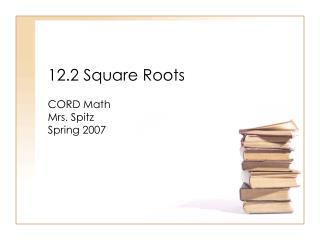Download Presentation12.2 Square Roots

# 12.2 Square Roots

Download Presentation## 12.2 Square Roots

- - - - - - - - - - - - - - - - - - - - - - - - - - - E N D - - - - - - - - - - - - - - - - - - - - - - - - - - -
##### Presentation Transcript

1. 12.2 Square Roots CORD Math Mrs. Spitz Spring 2007

2. Objectives • Simplify rational square roots, and • find approximate values for square roots

3. Assignment • pp. 479-480 #5-51 all

4. How to read square roots • 82 is read “eight squared and means eight is used as a factor two times. • -8 is used as a factor two times.

5. How to read square roots • The opposite of squaring a number is finding its square root. To find the square root of 64, you must find two EQUAL factors whose product is 64. • Since 8 times 8 is 64, one square root is 8. Since -8 times -8 is also 64, another square root of 64 is -8.

6. Definition of a Square Root • If x2 = y, then x is a square root of y.

8. What do these mean? indicate the principal square root of 64. indicates the negative square root of 64. indicates both square roots of 64. Note this is read, “plus or minus the square root of 64.”

9. Ex. 1: Find each square root. Since 92 = 81, you know that the square root is 9.

10. Ex. 2: Find each square root. Since (0.3)2 = 0.09, you know that the square root is ±0.3.

11. What if it doesn’t go evenly? • Sometimes you may need to use prime factorization to find a square root of a number.

12. Ex. 3: Find each square root. Since 242 = 576, you know that the negative square root is -24.

13. Ex. 4: Find each square root.

14. Calculators • If you happen to own one, and you should. I would highly recommend the TI-83 or 84 if you are going to continue in mathematics. If not, the TI30II-XS will suffice. It’s about \$15 at Wal-mart. In any case, you will need one if you are doing square roots or access to your textbook on page 645 which has a table of values.

15. Ex. 5. Find the square root of: Hit your 2nd button Square root – 1st row 6 buttons down. Enter the number 2209 and click enter or = The value should be 47.

16. Ex. 6. Find the square root of: Hit your 2nd button Square root – 1st row 6 buttons down. Enter the number 1236 and click enter or = The value should be 35.1567917